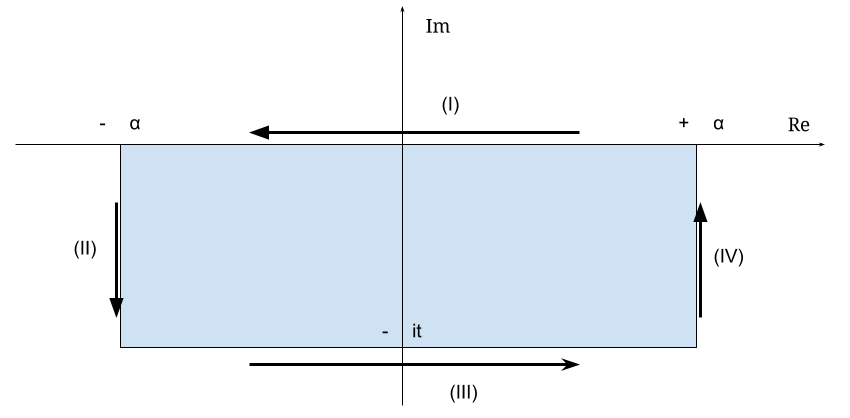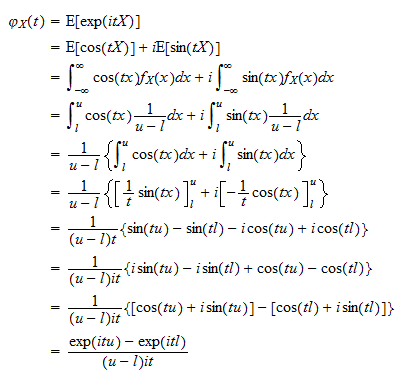From Wikipedia:

In probability theory and statistics, the characteristic function of any real-valued random variable completely defines its probability distribution. If a random variable admits a probability density function, then the characteristic function is the Fourier transform of the probability density function. Thus it provides the basis of an alternative route to analytical results compared with working directly with probability density functions or cumulative distribution functions.

$\color{blue}{\Large\varphi_X(t)=\mathbb E[e^{itX}]}$

#### STANDARD NORMAL DISTRIBUTION:

Now, the pdf of the standard normal ditribution is:

$f_X(X=x)=\frac{1}{\sqrt{2\pi}}e^{-\frac{x^2}{2}}$

Therefore, and by LOTUS:

\begin{align}\Large \varphi(t) &= \mathbb E[e^itX]\\ &= \int_{-\infty}^{+\infty} e^{itX} \frac{1}{\sqrt{2\pi}}e^{-\frac{x^2}{2}} dx\\ &=\int_{-\infty}^{+\infty} \color{blue}{\frac{e^{\frac{-t^2}{2}}}{\sqrt{2\pi}}e^{\frac{t^2}{2}}}e^{itx} e^{-\frac{x^2}{2}} dx\\ &=\frac{e^{\frac{-t^2}{2}}}{\sqrt{2\pi}}\int_{-\infty}^{+\infty}e^{\frac{t^2}{2}}e^{itx} e^{-\frac{x^2}{2}} dx\\ &=\frac{e^{\frac{-t^2}{2}}}{\sqrt{2\pi}}\int_{-\infty}^{+\infty}e^{-\frac{(x-it)^2}{2}} dx\\ \end{align}

Changing variables:

$s = x-it$

and

$ds = dx$

The limits of the integral will be:

$S_{\text{upper}}= \infty - it$

and

$S_{\text{lower}}= -\infty - it$

So we have:

$\varphi(t)=\frac{e^{\frac{-t^2}{2}}}{\sqrt{2\pi}}\int_{-\infty-it}^{+\infty-it}e^{-\frac{s^2}{2}} ds\tag 1$

To integrate this we need to know that the closed-loop or contour integral:

$\oint f(z) dz = 2\pi i \sum \text{Residuals}=0$

Provided that the function is analytic within the integration path in the complex plane:For the area in blue the function $$e^{-s^2/2}$$ is analytic: it can be expressed as a power series. Hence,

$\oint e^{-s^2/2}=0$

Integrating along (I) we are moving along the real line:

$\int_{\alpha}^{-\alpha}e^{-x^2/2}dx$

For part (II):

$\int_{-\alpha}^{\alpha-it}e^{-s^2/2}ds$

The third part (III):

$\int_{-\alpha-it}^{+\alpha-it}e^{s^2/2}ds$

The fourth part (IV):

$\int_{+\alpha-it}^{+\alpha}e^{s^2/2}ds$

So,

$0= \int_{\alpha}^{-\alpha}e^{-x^2/2}dx + \int_{-\alpha}^{\alpha-it}e^{-s^2/2}ds + \int_{-\alpha-it}^{+\alpha-it}e^{s^2/2}ds + \int_{+\alpha-it}^{+\alpha}e^{s^2/2}ds$

When $$\alpha \to \infty$$ we can see that the first term is the integral of the pdf of the standard normal without the normalizing factor, and integrating from plus to minus. Hence, it will evaluate to $$-\sqrt{2\pi}$$. The second and fourth parts evaluate to zero. So,

$0=-\sqrt{2\pi}+\int_{-\alpha-it}^{+\alpha-it}e^{s^2/2}ds$

or

$\sqrt{2\pi}= \int_{-\alpha-it}^{+\alpha-it}e^{s^2/2}ds$

And plugging this result into Eq. 1:

$\varphi(t)=\frac{e^{\frac{-t^2}{2}}}{\sqrt{2\pi}}\sqrt{2\pi} =e^{\frac{-t^2}{2}}$

### UNIFORM DISTRIBUTION:If $$t=0$$,

$\varphi(0)=\mathbb E[e^{i0x}]=\mathbb =1$

Hence, the characteristic function of the uniform distribution is:

$$\Large\varphi(t)=\frac{e^{itu}-e^{itl}}{(u-l)it}$$

when $$t\neq 0$$

and $$1$$ when it is $$0.$$

When the limits are $$-1$$ and $$1$$:

$$\Large\varphi(t)=\frac{e^{it}-e^{-it}}{2it}= \frac{1}{2it}$$